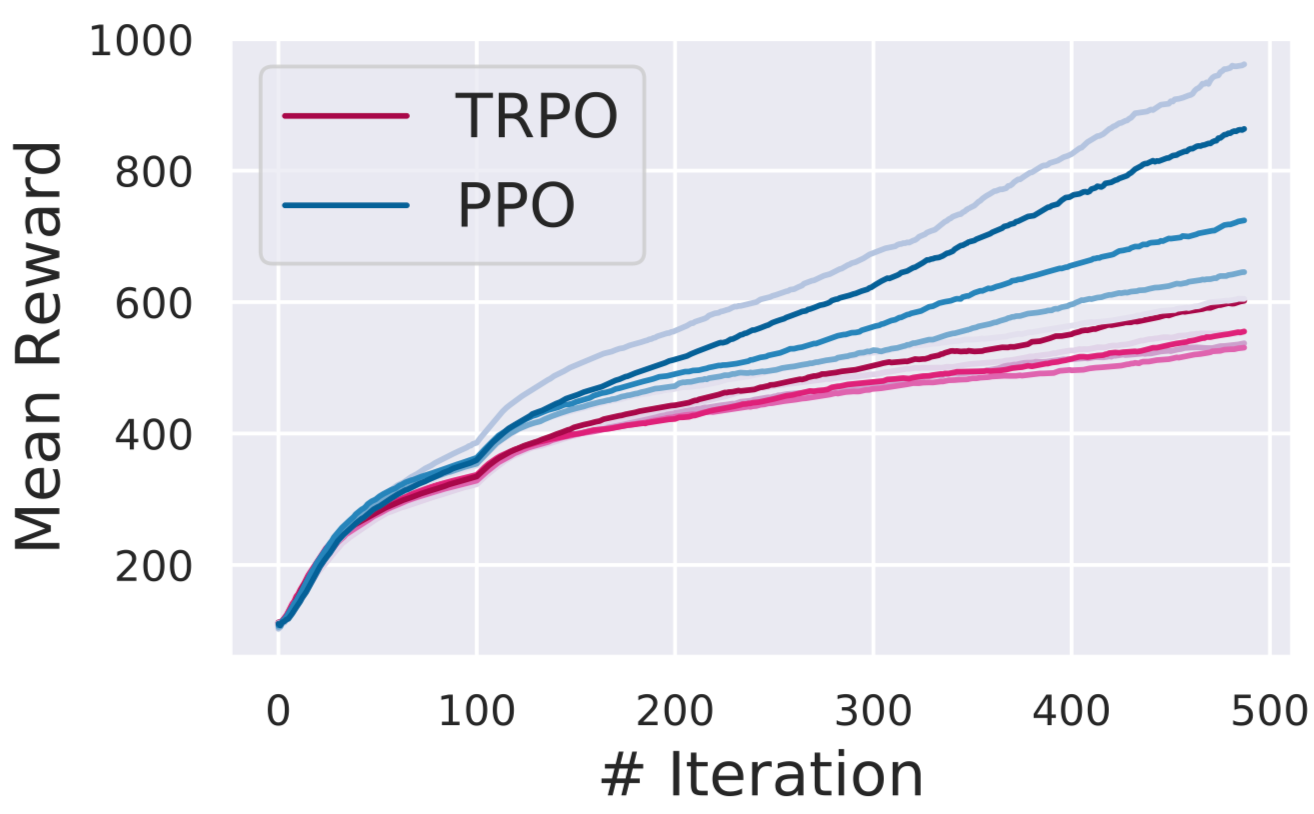# Proximal Policy Optimization(PPO)- A policy-based Reinforcement Learning algorithm

Original article was published by Dhanoop Karunakaran on Artificial Intelligence on Medium# Proximal Policy Optimization(PPO)- A policy-based Reinforcement Learning algorithm

Let’s dive into a few RL algorithms before discussing the PPO.

PPO is a policy gradient method where policy is updated explicitly. We can write the objective function or loss function of vanilla policy gradient with advantage function.

The main challenge of vanilla policy gradient RL is the high gradient variance. The standard approach to reduce the variance in gradient estimate is to use advantage function. The advantage function estimates how good an action compared to average action for a specific state. The idea here is to reduce the high variance in the gradient estimate between the old policy and the new policy. The reduction of variance helps to increase the stability of the RL algorithm. We can write the advantage function as below:

If the advantage function is positive, then it means action taken by the agent is good and we can a good reward by taking the action. So the idea here is to improve those actions probability. On other hand, if the advantage resulted in negative, then we need to decrease the action probabilities. The real problem is the advantage function can create a noisy estimate that can mess up the policy update. The reason is V(s) is another neural network and during the learning process, it can generate a noisy estimate for the given state due to the line search in gradine ascent.

Many Gradinet ascent method uses line search to find the global maxima for the optimisation task. In line search, we find the step size first, then determine the direction for the optimisation task. Sometime if the step size is large that lead to a negative direction it can cause damage to the learning process. Also, the small step size will result in lrager time to converge.

In trust region, we first decide the step size, α. We can construct a region by considering the α as the radius of the circle. We can call this region a trust region. The search for the best point(local minimum or local maximum in that region) for the improvement is bounded in that region. Once we have the best point it determines the direction. This process repeats until the optimal point is reached.

# Trust Region Policy Optimisation(TRPO)

In 2015, TRPO introduces trust region strategies to RL instead of the line search strategy. The TRPO add KL divergence constraints for enabling the trust-region for the optimisation process. It makes sure that the new updates policy is not far away from the old policy or we can say that the new policy is within the trust region of the old policy. It means policy update is not deviating largely. Please follow this link to know more about TRPO. We can write the objective function of TRPO.

We can interpret the equation as maximising the objective function subjected the satisfying the KL constraint term on the step size of the policy update.

TRPO is a relatively complicated algorithm. The KL constraint adds additional overhead in the form of hard constraints to the optimisation process. Also implementing the TRPO algorithm is not a straight forward task. Here comes the PPO which much simpler.

# Proximal Policy Optimization(PPO)

PPO is a first-order optimisation that simplifies its implementation. Similar to TRPO objective function, It defines the probability ratio between the new policy and old policy and we can call it as r(θ).

Now we can modify the objective function of TRPO as below:

Without adding constraints, this objective function can lead to instability or slow convergence rate due to large and small step size update respectively. Instead of adding complicated KL constraint, PPO imposes policy ratio, r(θ) to stay within a small interval around 1. That is the interval between 1-ϵ and 1+ϵ. ϵ is a hyperparameter and in the original PPO paper, it was set to 0.2. Now we can write the objective function of PPO.

In the above equation, the function clip truncates the policy ratio between the range [1-ϵ, 1+ϵ]. The objective function of PPO takes the minimum value between the original value and the clipped value. Similar to what we discussed in vanilla policy gradient section above, positive advantage function indicates the action taken by the agent is good. On the other hand, a negative advantage indicated bad action. For PPO, in both cases, the clipped operation makes sure it won’t deviate largely by clipping the update in the range.

We will talk about the implementation of PPO in a separate blog.

If you like my write up, follow me on Github, Linkedin, and/or Medium profile.